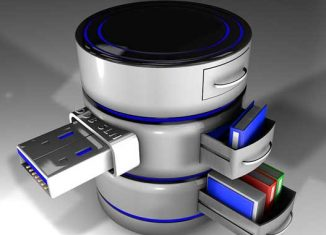# PHP MySQLi Create, Read, Update and Delete – 2In previous tutorial how to creating a connection, creating a database, creating a table using PHP MySQLi. Click here to view.

In this tutorial, I have to share how to

• Inserting data
• Updating data
• Deleting data
• Deleting a table
• Deleting a database

#### Inserting data

Syntax for the insert data in to a table.

`\$sql = " INSERT INTO table_name ( column_name1, column_name2, ... ) values('data1', 'data2', ... )";`

For inserting data in to a table as follow. In this example, I’ve insert ‘acc_name’, ‘acc_email’ and ‘password’ to the table ‘account’.

```<?php
\$dbhost = 'localhost';
\$dbuser = 'root';
\$dbpass = '';
\$conn = mysqli_connect(\$dbhost, \$dbuser, \$dbpass);
if(! \$conn )
die('Could not connect: ' . mysqli_error());
mysqli_select_db(\$conn,'test_db');
\$sql = " INSERT INTO account (acc_name, acc_email, password VALUES ( 'user1', 'contact@tricing.com', 'password123' ) ";
\$result = mysqli_query( \$conn, \$sql );
if(! \$result ) die('Could not enter data: ' . mysqli_error());
echo "Entered data successfully...";
mysqli_close(\$conn);
?>```

Syntax for reading data from a table

`\$sql = " SELECT column_name1, column_name2,... FROM tablename WHERE column_name = 'serch_string' ";`
##### SINGLE ROW

For retrieve single row from a table as follow. In this example, I’ve retrieve ‘acc_name’ and ‘acc_email’ from ‘account’ table where ‘acc_name’ is ‘user1’.

```<?php
\$dbhost = 'localhost';
\$dbuser = 'root';
\$dbpass = '';
\$conn = mysqli_connect(\$dbhost, \$dbuser, \$dbpass);
if(! \$conn )
die('Could not connect: ' . mysqli_error());
mysqli_select_db(\$conn,'test_db');
\$sql ="SELECT acc_name, acc_email FROM account WHERE acc_name LIKE 'user1'";
\$result = mysqli_query( \$conn, \$sql );
\$row = mysqli_fetch_array(\$result);
echo \$row.', '. \$row.' <br>';
echo \$row['acc_name'].', '. \$row['acc_email'].' <br>';
mysqli_close(\$conn);
?>```
##### MULTIPLE ROW

For retrieve multiple row from a table as follow. In this example, I’ve retrieve ‘acc_name’ and ‘acc_email’ from the ‘account’ table.

```<?php
\$dbhost = 'localhost';
\$dbuser = 'root';
\$dbpass = '';
\$conn = mysqli_connect(\$dbhost, \$dbuser, \$dbpass);
if(! \$conn )
die('Could not connect: ' . mysqli_error());
mysqli_select_db(\$conn,'test_db');
\$sql ="SELECT acc_name, acc_email FROM account ";
\$result = mysqli_query( \$conn, \$sql );
while(\$row = mysqli_fetch_array(\$result))
{
echo \$row.', '. \$row.' <br>';
echo '----------------------------------------------------<br>';
}
mysqli_close(\$conn);
?>```

#### Updating data

Syntax for updating data from a table.

```\$sql = " UPDATE table_name SET column_name1= 'data1', column_name2= 'data2', ...
WHERE column_name1= 'condition' AND column_name2= 'condition'..";```

For updating data from a table as follow. In this example, I’ve update ‘acc_email’ as ‘info@xxxx.com’ from ‘account’ table where ‘acc_name’ is ‘user1’.

```<?php
\$dbhost = 'localhost';
\$dbuser = 'root';
\$dbpass = '';
\$conn = mysqli_connect(\$dbhost, \$dbuser, \$dbpass);
if(! \$conn ) die('Could not connect: ' . mysqli_error());
mysqli_select_db(\$conn,'test_db');
\$sql = " UPDATE account SET acc_email ='info@xxxx.com' WHERE acc_name ='user1'";
\$result = mysqli_query( \$conn, \$sql );
if(! \$result ) die('Could not update: ' . mysqli_error());
echo "Updated successfully...";
mysqli_close(\$conn);
?>```

#### Deleting data

Syntax for deleting data from a table.

`\$sql = " DELETE FROM table_name WHERE column_name1= 'condition' AND column_name2= 'condition'..";`

For deleting data from a table as follow. In this example, I’ve delete a row from ‘account’ table where ‘acc_name’ is ‘user1’.

```<?php
\$dbhost = 'localhost';
\$dbuser = 'root';
\$dbpass = '';
\$conn = mysqli_connect(\$dbhost, \$dbuser, \$dbpass);
if(! \$conn ) die('Could not connect: ' . mysqli_error());
mysqli_select_db(\$conn,'test_db');
\$sql = " DELETE FROM account WHERE acc_name ='user1'";
\$result = mysqli_query( \$conn, \$sql );
if(! \$result ) die('Could not delete data: ' . mysqli_error());
else
echo "Deleted successfully.....";
mysqli_close(\$conn);
?>```

#### Deleting Table

Syntax for deleting a table.

`\$sql = " DROP TABLE table_name ";`

Example for deleting a table as follow. In this example, I’ve delete the table ‘account’ from the database ‘test_db’.

```<?php
\$dbhost = 'localhost';
\$dbuser = 'root';
\$dbpass = '';
\$conn = mysqli_connect(\$dbhost, \$dbuser, \$dbpass);
if(! \$conn )
die('Could not connect: ' . mysqli_error());
mysqli_select_db(\$conn,'test_db');
\$sql = " DROP TABLE account ";
\$result = mysqli_query( \$conn, \$sql );
if(! \$result ) die('Could not delete data: ' . mysqli_error());
echo "Deleted succssfully...";
mysqli_close(\$conn);
?>```

#### Deleting Database

Syntax for deleting a database

`\$sql = ' DROP DATABASE database_name ';`

Example for deleting a database as follow. In this example, I’ve delete the database ‘test_db’.

```<?php
\$dbhost = 'localhost';
\$dbuser = 'root';
\$dbpass = '';
\$conn = mysqli_connect(\$dbhost, \$dbuser, \$dbpass);
if(! \$conn ) die('Could not connect: ' . mysqli_error());
echo "Connected successfully<br>";
\$sql = ' DROP DATABASE test_db ';
\$db = mysqli_query( \$conn, \$sql );
if(! \$db ) die('Could not delete database: ' . mysqli_error());
echo "Database test_db deleted successfully...";
mysqli_close(\$conn);
?>```